Brainstorming Transporter 1- Grid Mapping

Arena Mapping Algorithm

To Demonstrate my grid we will be showing this matrix which is representing the nodes of the 5 x 5 grid.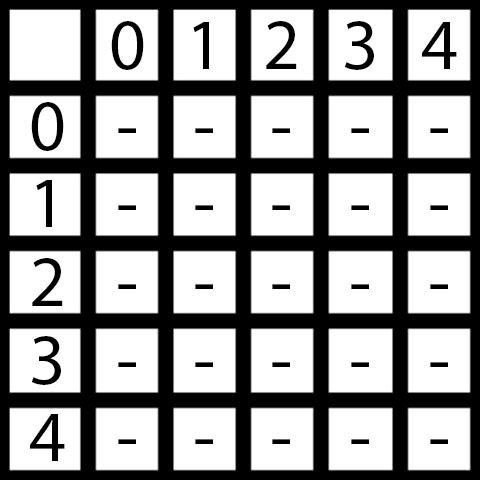``````“-” denotes that the arena at this node is unknown
“M” denotes that the arena at this node is marked (to be checked)
“1” denotes that the arena at this node is known and accessible
“0” denotes that the arena at this node is known and blocked
Initially my grid is totally unknown and so all the nodes are marked by dashes(“-”).
``````

The Algorithm:

This Algorithm is an extended version of BFS(Breadth First Search). We wont be teaching the BFS here but will describe it in brief here.

BFS: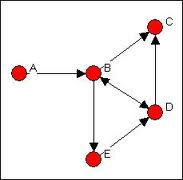Consider this graph as shown above.

(Graph: It is a set of nodes in which each and every node is connected to some other nodes. The connections are usually shown by an arrow. There can be multiple nodes pointing one node and a node can point multiple node)

Our problem is to search whether two nodes in the graph are connected or not. For this we have Breadth First Search:

For this we will create a Queue “Q” in which we will add and delete nodes

Enqueue the initial node to the queue Q.

Dequeue the front node and do the following steps: => If the node is already visited then jump to step 3 else mark the node as visited and move to next step. => After marking the current node as visited, enqueue all the nodes that are connected to this node(and not visited) in the queue.

If the queue is empty (All nodes connected with the first node are visited) then move to step 4 ,else jump to step 2.

Check if final node is visited: => If the final node is visited - “the final node and the initial node are connected”. => Else - “the final node and the initial node are not connected”.

Lets run this algorithm on the graph above:

``````Starting node “A”- Final node “D”
Q = {};//Initial Null Queue
//Enqueue Starting Node
Q = {“A”};
//Here our loop starts and iterates till the queue is not empty
//Dequeue the first Element, mark it visited and add the nodes connected to it
//First Iteration
Q.poll();//”A” is removed from queue
“A” = visited;
Q.add(“B”);//”B” is the only node connected to “A”
//Queue is not empty (Q = {“B”})
//Second Iteration
Q.poll();//”B” is removed from the queue
“B” = visited;
//”C” and “E” are added to the queue as they are directly connected to node “B”
//Queue is still not empty (Q = {“C”,”E”})
//Third Iteration
Q.poll();//”C” is removed from the queue
“C” = visited;
//no nodes are connected to “C”, therefore nothing is added to the queue
//Queue is still not empty (Q = {“E”})
//Fourth Iteration
Q.poll();//”E” is removed from the queue
“E” = visited;
Q.add(“D”)//only “D” is connected to “E”
//Queue is still not empty (Q = {“D”})
//Fifth Iteration
Q.poll();//”D” is removed from the queue
“D” = visited;
//”B” is directly connected to “D” but it won’t be added to the queue as it is already visited
//Queue is empty and therefore break the loop
//Check is “D” is visited
//Yes it is!!!
//Search successful
//BFS Complete
``````

Now for grid mapping a little advancement needs to be done in the previous algorithm. We will consider grid as a connected graph with each node directly connected to its four surrounding nodes(this may not be true for the nodes that lie on the border).Since we do not know the arena before hand and we need to traverse the grid we have to create a general algorithm that will tell the robot about the next node it has to reach.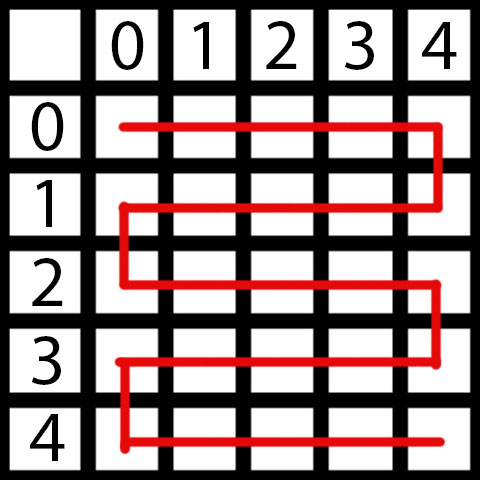The simplest algorithm to traverse a 5 x 5 grid can be as shown in the figure

This is not exactly a 5x5 grid but the matrix that stores the position of 25 nodes of the 5x5 grid

But the algorithm shown above wont work if the grid has some blocked nodes

Suppose my final Arena is this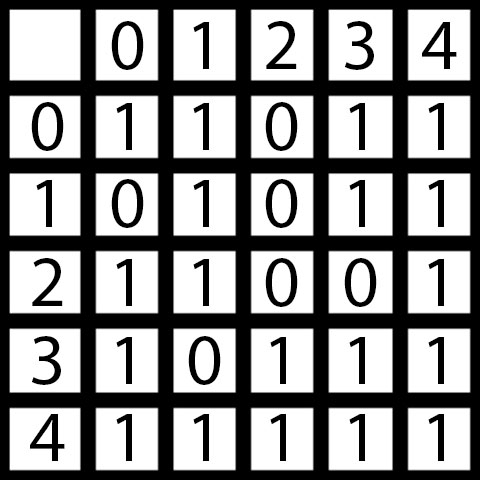Where “1” denotes the accessible node and “0” denotes the blocked node(either VOID or CUBE)

Here the simple algorithm wont work. Now lets try the BFS Approach to solve the GRID

Demostration:

``````“-” denotes that the arena at this node is unknown
“M” denotes that the arena at this node is marked (to be checked more specifically the nodes which are present in the queue)
“1” denotes that the arena at this node is known and accessible
“0” denotes that the arena at this node is known and blocked
``````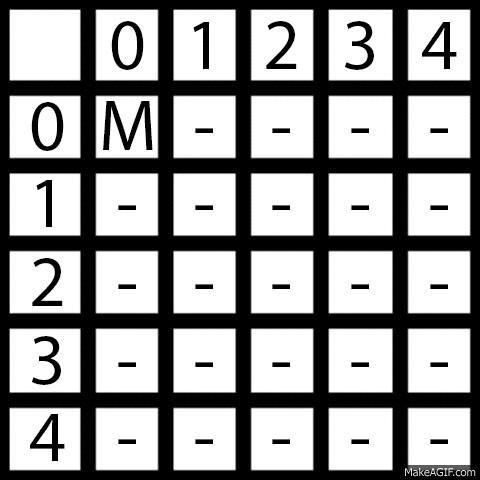Here we consider that blocked nodes connect no further nodes. So, when a blocked node arrives no extra node is further marked. Thus, we can traverse even an unknown grid using a variant of BFS.